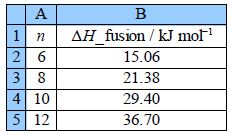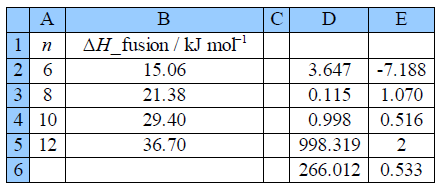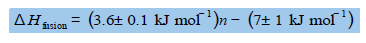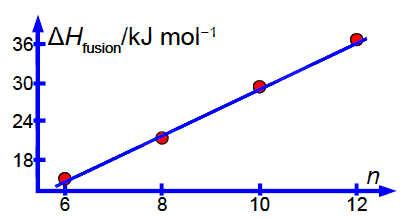# Enthalpy of Fusion of n-Carboxylic Acids?

Figure shows the enthalpy of fusion ∆H fusion of four linear saturated n-carboxylic acids as a function of n the number of carbon atoms in the molecule.Use LINEST to find the equation that correlates the enthalpy of fusion of the carboxylic acids with the number of C-atoms in their length and also find the precisions of the gradient and intercept. Organic chemists use this type of equation to correlate large tables of experimental data (not just the 4 pieces of data shown here). Finally draw a graph of the data with your LLSQ line shown.

Solution

Figure shows the final spreadsheet using LINEST:Taking account of the standard deviations, the fitted equation for the enthalphy variation is:Notice how the intercept is much less precise (14% relative precision) compared to the gradient (3%) so extrapolation is not recommended. However, with more data (such as from a large tabulation of data) the precision of the LLSQ correlation line would improve markedly and become really useful in organic chemistry. Also with more data you would find that you need two slightly different linear equations for n with even and odd numbers of C-atoms. Finally we should always check our results by plotting a graph to see if any of the points are rogue ones and to confirm that the fitted line is sensible below Figure.